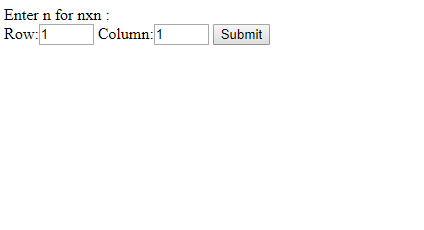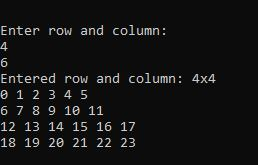GFG App
Open AppBrowser
Continue

# How to take user input for two dimensional (2D) array in PHP ?

There are two methods to take user input in PHP in two dimensional (2D) array.

Approach 1:

• Use HTML forms through PHP GET & POST method to take user input in two dimensional (2D) array.
• First, input data into HTML forms.
• Then use the GET or POST method of PHP to get or post those input data into a variable.
• Finally, use that variable that holds input data and process using for loop.
• Though it is from a two-dimensional array so, you need two indices/variables for processing in for loop.
• Enter input one below another to state as two variables.

Example 1: Below example illustrate how to input user data for 2D-array using the Form POST method.

## php

 `"``;` `echo` `"
` `    ``Row:` `    ``Column:` `    ``` `";`   `// Submit user input data for 2D array` `if` `(isset(``\$_POST``[``'submit'``])) {` `    `  `    ``// POST submitted data` `    ``\$dimention1` `= ``\$_POST``[``"1d"``];` `    `  `    ``// POST submitted data` `    ``\$dimention2` `= ``\$_POST``[``"2d"``];` `    `  `    ``echo` `"Entered 2d nxn: "` `. ``\$dimention1` `            ``. ``"x"` `. ``\$dimention2` `. ``"
"``;` `    ``\$d` `= [];` `    ``\$k` `= 0;` `    `  `    ``for``(``\$row` `= 0; ``\$row` `< ``\$dimention1``; ``\$row``++) {` `        ``for` `(``\$col` `= 0; ``\$col` `< ``\$dimention2``; ``\$col``++) {` `            ``\$d``[``\$row``][``\$col``]= ``\$k``++;` `        ``}` `    ``}` `    `  `    ``for` `(``\$row` `= 0; ``\$row` `< ``\$dimention1``; ``\$row``++) {` `        ``for` `(``\$col` `= 0; ``\$col` `< ``\$dimention2``; ``\$col``++) {` `            ``echo` `\$d``[``\$row``][``\$col``].``" "``;` `        ``}` `        ``echo` `"
"``;` `    ``}` `}` `?>`

Output:Approach 2:

• To take user input in PHP for two dimensional (2D) by using fopen() function which helps to get user input either at runtime or by the external input file.
• First, assign those input data to variables.
• Finally, use those variables which hold input data and process using for loop.
• Though it is from a two-dimensional array so, you need two indices/variables for processing in for loop.

Example: Below example illustrates how to input user data for a 2D array using fopen() function.

## php

 `` ``

Output:My Personal Notes arrow_drop_up